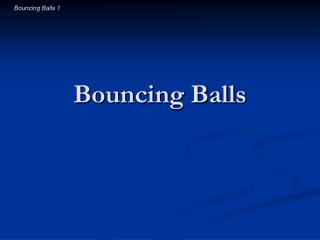DownloadDownload PresentationBouncing Balls

# Bouncing Balls

Download Presentation## Bouncing Balls

- - - - - - - - - - - - - - - - - - - - - - - - - - - E N D - - - - - - - - - - - - - - - - - - - - - - - - - - -
##### Presentation Transcript

1. Bouncing Balls

2. Introductory Question • If you place a tennis ball on a basketball and drop this stack on the ground, how high will the tennis ball bounce? • To approximately its original height • Much higher than its original height • Much less than its original height

3. Observations aboutBouncing Balls • Some balls bounce better than others • Dropped balls don’t rebound to their full height • Balls bounce differently from different surfaces • Ball bounce differently from moving objects

4. 4 Questions about Bouncing Balls • Why can’t a ball that’s dropped on a hard floor rebound to its starting height? • Why does the floor’s surface affect the bounce? • How does a ball bounce when it hits a bat? • What happens to the bat when a ball hits it?

5. Question 1 • Why can’t a ball that’s dropped on a hard floor rebound to its starting height? • What happens to ball’s energy as it bounces?

6. Bouncing from a Rigid Floor • As it strikes a rigid floor, a ball’s • kinetic energy decreases by the “collision” energy • elastic potential energy increases as it dents • As it rebounds from that surface, the ball’s • elastic potential energy decreases as it undents • kinetic energy increases by the “rebound” energy • Rebound energy < collision energy • A “lively” ball wastes little energy as thermal energy • A “dead” ball wastes most of its energy

7. Measuring a Ball’s Liveliness • Coefficient of Restitution • is a measure of a ball’s liveliness • is the ratio of outgoing to incoming speeds: coefficient of restitution = outgoing speed / incoming speed

8. Question 2 • Why does the floor’s surface affect the bounce?

9. Bouncing from an Elastic Floor • Both ball and floor dent during a bounce • Work is proportional to dent distance • Denting floor stores and returns energy • A “lively” floor wastes little energy • A “dead” floor wastes most of its energy • A floor has a coefficient of restitution, too • A soft, lively floor can help the ball bounce!

10. Question 3 • How does a ball bounce when it hits a bat? • Do both the ball and bat bounce?

11. Bouncing from Moving Surfaces • Incoming speed → approaching speed • Outgoing speed → separating speed • Coefficient of Restitution becomes: coefficient of restitution = separating speed / approaching speed

12. Ball and Bat (Part 1) • Ball heads toward home plate at 100 km/h • Bat heads toward pitcher at 100 km/h • Approaching speed is 200 km/h

13. Ball and Bat (Part 2) • Approaching speed is 200 km/h • Baseball’s coefficient of restitution: 0.55 • Separating speed is 110 km/h

14. Ball and Bat (Part 3) • Separating speed is 110 km/h • Bat heads toward pitcher at 100 km/h • Ball heads toward pitcher at 210 km/h

15. Introductory Question (revisited) • If you place a tennis ball on a basketball and drop this stack on the ground, how high will the tennis ball bounce? • To approximately its original height • Much higher than its original height • Much less than its original height

16. Question 4 • What happens to the bat when a ball hits it?

17. Bouncing’s Effects on Objects • A bouncing ball transfers momentum • while stopping • while rebounding • A livelier ball transfers more momentum • A bouncing ball can also transfer energy • These two transfers together govern bouncing • A ball transfers momentum and energy to a bat • Identical elastic balls can transfer motion perfectly

18. Impact Forces • Harder surfaces bounce faster • Momentum is transferred more quickly • Time is shorter, so force is larger • No one likes bouncing off hard surfaces

19. The Ball’s Effects on a Bat • The ball pushes the bat back and twists it, too • When the ball hits the bat’s center of percussion, • the bat’s backward and rotational motions balance • the bat’s handle doesn’t jerk • When the ball hits the bat’s vibrational node, • the bat doesn’t vibrate • more energy goes into the ball

20. Summary about Bouncing Balls • Each ball has a coefficient of restitution • Energy lost in a bounce becomes thermal • The surface can affect a ball’s bounce • Surfaces bounce, too